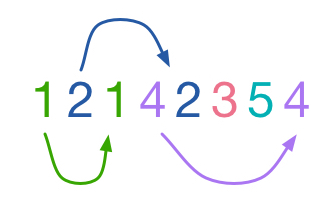# Arrangement

Probability Level 2Is it possible to arrange the 3972 numbers $1, 1, 2, 2, 3, 3, \ldots , 1986, 1986$ into one line such that there are exactly $n$ numbers between any two $n$'s?

×